## The SpaceRn

By analogy with the preceding constructions (R 2 and R3), you can consider the collection of all ordered n‐tuples of real numbers (x 1, x 2, …, x n) with the analogous operations of addition and scalar multiplication. This is called n‐space (denoted R n ), and vectors in R n are called n‐vectors . The standard basis vectors in R n arewhere e k has a 1 in the kth place and zeros elsewhere. All the figures above depicted points and vectors in R 2 and R 3. Although it is not possible to draw such diagrams to illustrate geometric figures in R n if n > 3, it is possible to deal with them algebraically, and therein lies the real power of the algebraic machinery.

Example 1: Consider the vectors a = (1, 2, −3), b = (0, 1, −4, 2), and c = (5, −1, −1, 1) in R 4. Determine the vector 2 ab + c.

Extend the definitions of scalar multiplication and vector addition in the natural way to vectors in R 4 to compute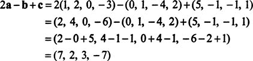Example 2: Determine the sum of the standard basis vectors e 1, e 3, and e 4 in R 5.

All vectors in R 5 have five components. Four of the components in each of the standard basis vectors in R 5 are zero, and one component—the first in e 1, the third in e 3, and the fourth in e 4—has the value 1. Therefore,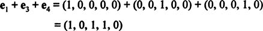The norm of a vector. The length (or Euclidean norm) of a vector x is denoted ‖ x‖, and for a vector x = (x 1, y2) in R 2, ‖ x‖ is easy to compute (see Figure ) by applying the Pythagorean Theorem:Figure 1

The expression for the length of a vector x = (x 1, x 2, x3) in R 3 follows from two applications of the Pythagorean Theorem, as illustrated in Figure :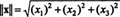Figure 2

In general, the norm of a vector x = (x 1, x 2, x 3,…, x n) in R n is given by the equationExample 3: The length of the vector x = (3, 1, −5, 1) in R 4 isExample 4: Let x be a vector in R n. If c is a scalar, how does the norm of c x compare to the norm of x?

If x = (x1, x2,…, x n), then c x = (cx1, cx2,…, cxn). Therefore,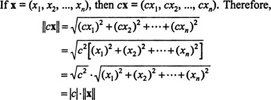Thus, multiplying a vector by a scalar c multiplies its norm by | c|. Note that this is consistent with the geometric description given earlier for scalar multiplication.

Distance between two points. The distance between two points x and y in R n —a quantity denoted by d(x, y)—is defined to be the length of the vector xy:Example 5: What is the distance between the points p = (3, 1, 4) and q = (1, 3, 2)?

Since pq = q − p = (1, 3, 2) − (3, 1, 4) = (−2, 2, −2), the distance between the points p and q is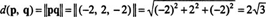Unit vectors. Any vector whose length is 1 is called a unit vector. Let x be a given nonzero vector and consider the scalar multiple x/‖ x‖. (The zero vector must be excluded from consideration here, for if x were 0, then ‖ x‖ would be 0, and the expression x/‖ x‖ would be undefined.) Applying the result of Example 4 (with c = 1/‖ x‖), the norm of the vector x/‖ x‖ is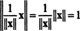Thus, for any nonzero vector x,is a unit vector. This vector is denoted (“x hat”) and represents the unit vector in the direction of x. (Indeed, one can go further and actually call the direction of x.) Note in particular that all the standard basis vectors are unit vectors; they are sometimes written as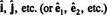to emphasize this fact.

Example 6: Find the vector y in R 2 whose length is 10 and which has the same direction as x = 3 i + 4 j.

The idea is simple: Find the unit vector in the same direction as 3 i + 4 j, and then multiply this unit vector by 10. The unit vector in the direction of x is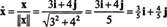Therefore,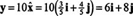The dot product. One way to multiply two vectors—if they lie in R 3—is to form their cross product. Another way to form the product of two vectors—from the same space R n , for any n—is as follows. For any two n‐vectors x = (x1, x2,…, xn) and y = (y1, y2,…, yn), their dot product (or Euclidean inner product) is defined by the equation(The symbol x·y is read “x dot y.”) Note carefully that, unlike the crooss product, the dot product of two vectors is a scalar. For this reason, the dot product is also called the scalar product. It can be easily shown that the dot product on R n satisfies the following useful identities: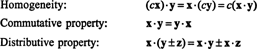Example 7: What is the dot product of the vectors x=(−1, 0, 4) and y =(3, 6, 2) in R 3?

By the commutative property, it doesn't matter whether the product is taken to be x·y or y·x; the result is the same in either case. Applying the definition yieldsThe dot product of a vector x= (x 1, x 2,…, x n) with itself is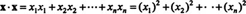Notice that the right‐hand side of this equation is also the expression for ‖ x2: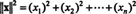Therfore, for any vector xThis identity is put to use as follows. Since ‖ a2 = a·a, the distributive and commutative properties of the dot product imply that for any vectors x and y in R n ,Thus, ‖x + y ‖ 2 = ‖x‖ 2 + 2 x· y + ‖y‖ 2 (*)

Now, if xy, then by figure , the Pythagorean Theorem would sayFigure 3

Therefore, if xy, equation (*) and (**) implywhich simplifies to the simple statement x·y = 0. Since this argument is reversible (assuming that it is agreed that the zero vector is orthogonal to every vector), the following fact has been established: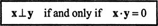This says that two vectors are orthogonal—that is, perpendicular—if and only if their dot product is zero.

Example 8: Use the dot product to verify that the cross product of the vectors x = (2,3,0) and y =(−1,1,4) is orthogonal to both x and y; then show that x x y is orthogonal to both x and y for any vectors x and y in R 3.

You can determine that x x y =(12,−8,5). The criterion for orthogonality is the vanishing of the dot product. Since bothand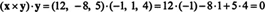the vector x x y is indeed orthogonal to x and to y. In general,and a similar calculation shows that (x x yx = 0 also.

The triangle inequality. From elementary geometry, you know that the sum of the lengths of any two sides of a triangle must be greater than the length of the third side. That is, if A, B, and C are the vertices of a triangle, then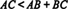This just says that the direct journey from A to C (along side AC) is shorter than the path from A to B and then to C; see Figure . This is called the triangle inequality.Figure 4

The triangle inequality can be generalized to vectors in R n . If x and y are any two n‐vectors, then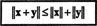Figure shows that this statement can be interpreted in the same way as the elementary geometric fact about the lengths of the sides of a triangle. [One notable difference, however, is that if x and y happen to be parallel (that is, if y is a positive scalar times x) or if either x or y is the zero vector, then ‖ x + y‖ = ‖ x‖ + ‖ y‖. The generalized triangle inequality must take these degenerate cases into account (hence the weak inequality, ≤), whereas the triangle inequality from elementary geometry does not (and hence the strong (or strict) inequality, <).]Figure 5

Example 9: Verify the triangle inequality for the vectors x= (−1, 0, 4) and y =(3, 6, 2) from Example 20.

The sum of these vectors is x + y = (2, 6, 6), and the lengths of the vectors x, y, and x + y are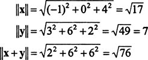With these lengths, the triangle inequality, ‖ x + y‖≤‖ x‖+‖ y‖, becomes √76 ≤ √17+7, which is certainly true, since the lefthand side is less than 9, while the right‐hand side is greater than 4 + 7 = 11.

The Cauchy‐Schwarz inequality. One of the most important inequalities in mathematics is known as the Cauchy‐Schwarz inequality. For R n equipped with an inner product, this inequality stateswhich says the absolute value of the dot product of two vectors is never greater than the product of their norms. Because of this inequality, it must be true that for any two nonzero vectors x and y,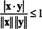Since both ‖ x‖ and ‖ y‖ are positive, the absolute value signs can be repositiones: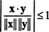a statement which now directly impliesThis final inequality says that there is precisely one value of θ between 0 and π (inclusive) such that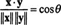This θ is called the angle between the vectors x and y; geometrically, it is the smaller angle between them. (Note: No angle θ is defined if either x or y is the zero vector.) To verify that this θ is indeed the geometric angle between x and y, consider Figure , where it is assumed that the angle between x and y is acute.Figure 6

The vector z is orthogonal to x, and the figure shows that y is the sum of z and a positive scalar multiple, c x, of x: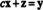Taking the dot product of both sides of this equation with x yieldsSince x and z are orthogonal, the dot product x·z is 0. This reduces the equation above to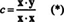But Figure and the definition of cos θ indicate thatNow, since c is positive, ‖ c x‖ = | c| ‖ x‖ = cx‖, so this equation becomes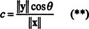Equations (*) and (**), together with the identity x· x, then imply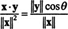which becomes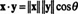This proof can be extended to the case where the angle between x and y is obtuse, thus validating the following alternate—but entirely equivalent—definition of the dot product:Note that this equation is consistent with the observation xyx· y = 0, since θ = π/2 implies cosθ = 0.

Example 10: Use the dot product to determine the angle between the vectors x = (2, 3, 0) and y = (−1, 1, 4).

From the boxed equation directly above,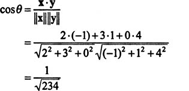Therefore, θ = cos −1 √1/234.

[Note that θ = sin −1 √233/234. Although this is consistent with the present calculation (because cos 2 θ + sin 2 θ must always equal 1 for any θ, and this is certainly true here), it is better to use the dot product than the cross product to determine the angle between two vectors in R 3. Why? The statement sinθ = √233/234 implies that θ is either 86.25 ° or 93.75 °, and without further investigation, it is difficult to say which is correct. Even a picture may not help here; the angle is so close to 90 ° that your drawing will probably not be accurate enough to tell the difference. But the statement cosθ = √1/234 says that θ is definitely 86.25 °, with no ambiguity. Within the range between 0 and 180 °, the sine function is entirely positive and cannot differentiate between an acute angle and its supplement. However, the cosine function is positive for acute angles and negative for obtuse angles, so it can—immediately—differentiate between an acute angle and its supplement.]

Orthogonal projections. Consider two nonzero vectors x and y emanating from the origin in R n . Dropping a perpendicular from the tip of x to the line containing y gives the (orthogonal) projection of x onto y. This vector is denoted proj yx .

If θ < π/2 (Figure ), then the component of x along y, a positive scalar denoted comp y x, is equal to the norm of the (vector) projection of x onto y. If θ > π/2 (Figure ), then the component of x along y is a negative scalar, equal to the negative of the norm of the projection of x onto y. (And if θ = π/2, then comp y x = 0, since the orthogonal projection of x onto y is the zero vector.) In any case, the following equation holds:where θ is the angle between x and y. Now, since x · y = ‖ x‖ ‖ y‖cosθ, this equation for the component of x along y can be rewritten as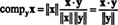Figure 7Figure 8

The vector projection of x onto y is equal to this scalar times the unit vector in the direction of yOr, since ‖ y2 = y·y,Example 11: Find the projection of x = (2, 2, 4) onto the vector y = (2, 6, 3).

If θ is the angle between x and y, then the component of x along y is given byTherefore,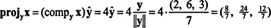See Figure .Figure 9

Lines. A line is determined by two distinct points, say p and q. If the vector pq is drawn from p to q, then pq defines the line's direction. The description of a line can therefore be reformulated as follows: A line L is uniquely determined by

a given point through which L passes,

and

a given (nonzero) vector which is parallel to L

Let p be the given point through which the line will pass, and let v be the vector that defines its direction.

From Figure , it is easy to see that a point x will be on the line if and only if the vector px is parallel (or antiparallel) to v, which happens if px is a scalar multiple of v:Figure 10

Or, since px = x − p,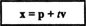This is the parametric equation for the line through p parallel to v. The scalar t is the parameter, and every point on the line is given by a particular choice of t.

Example 12: Find the equation of the line L in R 3 that passes through the point p = (2, 4, 2) and is parallel to the vector v = (1, 2, 3). Where does this line pierce the x−y plane?

A point x = ( x, y, z) is on the line L if and only if the vector px is a scalar multiple of v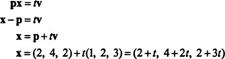Therefore,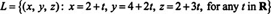Now, the line intersects the x−y plane when z = 0. SinceL pierces the x−y plane when the parameter t takes on the value −2/3. For this t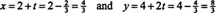so the point of intersection of L and the x−y plane is a = (4/3, 8/3, 0). See Figure .Figure 11

Example 13: Give the equation of the line in R 4 that passes through the points a = (−1, 1, 2, 0) and b = (3, 4, 0, −5). Does the point c = (7, 7, −2, −2) lie on this line?

Since the line is parallel to the vectorevery point x on the line is described by the parametric equation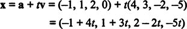The point c = (7, 7, −2, −2) will lie on this line if and only if there is a value of the parameter t such that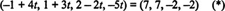However, although the first three components in (*) agree when t = 2, the fourth components do not. Therefore, the point c does not lie on the line.

Planes. A plane in R 3 is determined by three noncollinear points. If these points are labeled a, b, and c, then the cross product of the vectors ab and ac will give a vector v perpendicular to the plane. This vector v defines the plane's orientation in space; see Figure .Figure 12

The description of a plane can be formulated as follows: A plane P is uniquely determined by

• a given point through which P passes,

and

• a given nonzero vector which is normal—that is, perpendicular—to P

Let a be the given point on the plane, and let v be the defining normal vector, with its initial point at a. Then, as illustrated in Figure , for a point x to lie in P, v must be perpendicular to the vector ax.Figure 13

Since vaxv · ax = 0, the plane is determined by the equation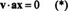To illustrate, let the point a = ( a 1, a 2, a 3) and the vector v = ( v 1, v 2, v 3). Since for any point x = ( x, y, z), the vector ax = x − a = ( x − a 1, y − a 2, z − a 3 ), equation (*) becomeswhich can also be written aswhere d = v 1 a 1 + v 2 a 2 + v 3 a 3. For a plane in R 3, this is the standard equation. Note carefully that the coefficients of x, y, and z in the standard equation are precisely the components of a vector normal to the plane.

Example 14: Give the standard equation of the plane P determined by the points p = (2, −1, 2), q = (2, 2, −1), and r = (0, 1, 1). Does this plane contain the origin? If not, give the equation of the plane which is parallel to P that does contain the origin.

The vectors pq = (0, 3, −3) and pr = (−2, 2, −1) lie in P, and their cross product, pq x pr, is normal to P. See Figure .Figure 14

Recalling the expression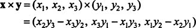for the cross product, it is determined thatSince the vector v = (3, 6, 6) is normal to P, the standard equation of P is given by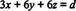for some constant d. Substituting the coordinates of any of the three given points ( p, q, or r) into this equation yields d = 12. Thus, P is given by the equation 3 x + 6 y + 6 z = 12, or more simply,Now, since (0, 0, 0) does not satisfy this equation, P does not contain the origin. However, the equation x + 2 y + 2 z = 0 specifies a plane parallel to P which is satisfied by 0 = (0, 0, 0). See Figure .Figure 15